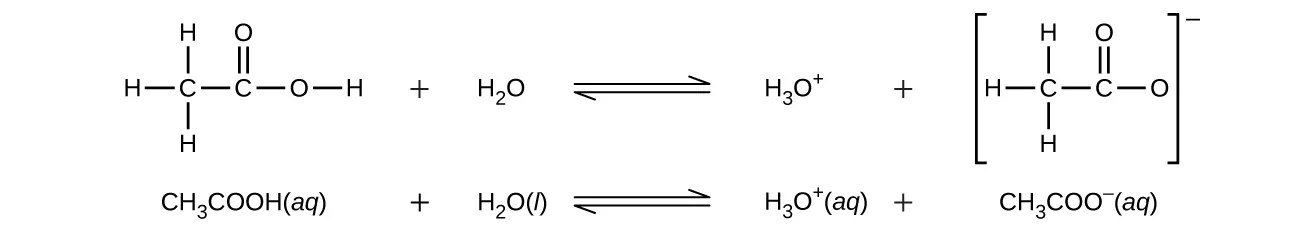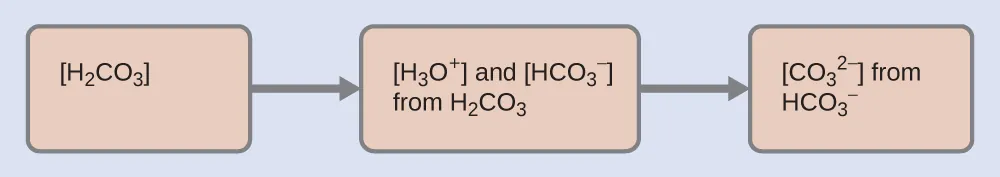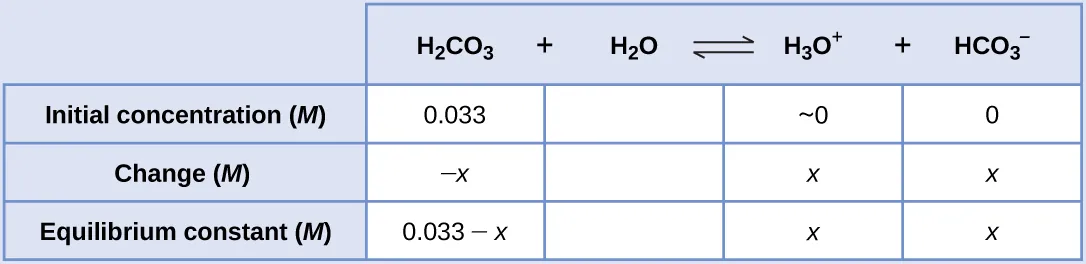Chemistry: Atoms First

# 14.5Polyprotic Acids

Chemistry: Atoms First14.5 Polyprotic Acids

### Learning Objectives

By the end of this section, you will be able to:
• Extend previously introduced equilibrium concepts to acids and bases that may donate or accept more than one proton

We can classify acids by the number of protons per molecule that they can give up in a reaction. Acids such as HCl, HNO3, and HCN that contain one ionizable hydrogen atom in each molecule are called monoprotic acids. Their reactions with water are:

$HCl(aq)+H2O(l)⟶H3O+(aq)+Cl−(aq)HNO3(aq)+H2O(l)⟶H3O+(aq)+NO3−(aq)HCN(aq)+H2O(l)⇌H3O+(aq)+CN−(aq)HCl(aq)+H2O(l)⟶H3O+(aq)+Cl−(aq)HNO3(aq)+H2O(l)⟶H3O+(aq)+NO3−(aq)HCN(aq)+H2O(l)⇌H3O+(aq)+CN−(aq)$
14.149

Even though it contains four hydrogen atoms, acetic acid, CH3CO2H, is also monoprotic because only the hydrogen atom from the carboxyl group (COOH) reacts with bases:Similarly, monoprotic bases are bases that will accept a single proton.

Diprotic acids contain two ionizable hydrogen atoms per molecule; ionization of such acids occurs in two steps. The first ionization always takes place to a greater extent than the second ionization. For example, sulfuric acid, a strong acid, ionizes as follows:

$First ionization:H2SO4(aq)+H2O(l)⇌H3O+(aq)+HSO4−(aq)Ka1=more than102; complete dissociationSecond ionization:HSO4−(aq)+H2O(l)⇌H3O+(aq)+SO42−(aq)Ka2=1.2×10−2First ionization:H2SO4(aq)+H2O(l)⇌H3O+(aq)+HSO4−(aq)Ka1=more than102; complete dissociationSecond ionization:HSO4−(aq)+H2O(l)⇌H3O+(aq)+SO42−(aq)Ka2=1.2×10−2$
14.150

This stepwise ionization process occurs for all polyprotic acids. When we make a solution of a weak diprotic acid, we get a solution that contains a mixture of acids. Carbonic acid, H2CO3, is an example of a weak diprotic acid. The first ionization of carbonic acid yields hydronium ions and bicarbonate ions in small amounts.

$First ionization:H2CO3(aq)+H2O(l)⇌H3O+(aq)+HCO3−(aq)KH2CO3=[H3O+][HCO3−][H2CO3]=4.3×10−7First ionization:H2CO3(aq)+H2O(l)⇌H3O+(aq)+HCO3−(aq)KH2CO3=[H3O+][HCO3−][H2CO3]=4.3×10−7$
14.151

The bicarbonate ion can also act as an acid. It ionizes and forms hydronium ions and carbonate ions in even smaller quantities.

$Second ionization:HCO3−(aq)+H2O(l)⇌H3O+(aq)+CO32−(aq)KHCO3−=[H3O+][CO32−][HCO3−]=5.6×10−11Second ionization:HCO3−(aq)+H2O(l)⇌H3O+(aq)+CO32−(aq)KHCO3−=[H3O+][CO32−][HCO3−]=5.6×10−11$
14.152

$KH2CO3KH2CO3$ is larger than $KHCO3−KHCO3−$ by a factor of 104, so H2CO3 is the dominant producer of hydronium ion in the solution. This means that little of the $HCO3−HCO3−$ formed by the ionization of H2CO3 ionizes to give hydronium ions (and carbonate ions), and the concentrations of H3O+ and $HCO3−HCO3−$ are practically equal in a pure aqueous solution of H2CO3.

If the first ionization constant of a weak diprotic acid is larger than the second by a factor of at least 20, it is appropriate to treat the first ionization separately and calculate concentrations resulting from it before calculating concentrations of species resulting from subsequent ionization. This can simplify our work considerably because we can determine the concentration of H3O+ and the conjugate base from the first ionization, then determine the concentration of the conjugate base of the second ionization in a solution with concentrations determined by the first ionization.

### Example 14.19

#### Ionization of a Diprotic Acid

When we buy soda water (carbonated water), we are buying a solution of carbon dioxide in water. The solution is acidic because CO2 reacts with water to form carbonic acid, H2CO3. What are $[H3O+],[H3O+],$ $[HCO3−],[HCO3−],$ and $[CO32−][CO32−]$ in a saturated solution of CO2 with an initial [H2CO3] = 0.033 M?
$H2CO3(aq)+H2O(l)⇌H3O+(aq)+HCO3−(aq)Ka1=4.3×10−7H2CO3(aq)+H2O(l)⇌H3O+(aq)+HCO3−(aq)Ka1=4.3×10−7$
14.153
$HCO3−(aq)+H2O(l)⇌H3O+(aq)+CO32−(aq)Ka2=5.6×10−11HCO3−(aq)+H2O(l)⇌H3O+(aq)+CO32−(aq)Ka2=5.6×10−11$
14.154

#### Solution

As indicated by the ionization constants, H2CO3 is a much stronger acid than $HCO3−,HCO3−,$ so H2CO3 is the dominant producer of hydronium ion in solution. Thus there are two parts in the solution of this problem: (1) Using the customary four steps, we determine the concentration of H3O+ and $HCO3−HCO3−$ produced by ionization of H2CO3. (2) Then we determine the concentration of $CO32−CO32−$ in a solution with the concentration of H3O+ and $HCO3−HCO3−$ determined in (1). To summarize:1. Step 1.
Determine the concentrations of $H3O+H3O+$ and $HCO3−.HCO3−.$
$H2CO3(aq)+H2O(l)⇌H3O+(aq)+HCO3−(aq)Ka1=4.3×10−7H2CO3(aq)+H2O(l)⇌H3O+(aq)+HCO3−(aq)Ka1=4.3×10−7$
14.155

As for the ionization of any other weak acid:An abbreviated table of changes and concentrations shows:Substituting the equilibrium concentrations into the equilibrium gives us:
$KH2CO3=[H3O+][HCO3−][H2CO3]=(x)(x)0.033−x=4.3×10−7KH2CO3=[H3O+][HCO3−][H2CO3]=(x)(x)0.033−x=4.3×10−7$
14.156

Solving the preceding equation making our standard assumptions gives:
$x=1.2×10−4x=1.2×10−4$
14.157

Thus:
$[H2CO3]=0.033M[H2CO3]=0.033M$
14.158

$[H3O+]=[HCO3−]=1.2×10−4M[H3O+]=[HCO3−]=1.2×10−4M$
14.159
2. Step 2.
Determine the concentration of $CO32−CO32−$ in a solution at equilibrium with $[H3O+][H3O+]$ and $[HCO3−][HCO3−]$ both equal to 1.2 $××$ 10−4 M.
$HCO3−(aq)+H2O(l)⇌H3O+(aq)+CO32−(aq)HCO3−(aq)+H2O(l)⇌H3O+(aq)+CO32−(aq)$
14.160

$KHCO3−=[H3O+][CO32−][HCO3−]=(1.2×10−4)[CO32−]1.2×10−4KHCO3−=[H3O+][CO32−][HCO3−]=(1.2×10−4)[CO32−]1.2×10−4$
14.161

$[CO32−]=(5.6×10−11)(1.2×10−4)1.2×10−4=5.6×10−11M[CO32−]=(5.6×10−11)(1.2×10−4)1.2×10−4=5.6×10−11M$
14.162

To summarize: In part 1 of this example, we found that the H2CO3 in a 0.033-M solution ionizes slightly and at equilibrium [H2CO3] = 0.033 M; $[H3O+][H3O+]$ = 1.2 $××$ 10−4; and $[HCO3−]=1.2×10−4M.[HCO3−]=1.2×10−4M.$ In part 2, we determined that $[CO32−]=5.6×10−11M.[CO32−]=5.6×10−11M.$

The concentration of H2S in a saturated aqueous solution at room temperature is approximately 0.1 M. Calculate $[H3O+],[H3O+],$ [HS], and [S2−] in the solution:
$H2S(aq)+H2O(l)⇌H3O+(aq)+HS−(aq)Ka1=8.9×10−8H2S(aq)+H2O(l)⇌H3O+(aq)+HS−(aq)Ka1=8.9×10−8$
14.163
$HS−(aq)+H2O(l)⇌H3O+(aq)+S2−(aq)Ka2=1.0×10−19HS−(aq)+H2O(l)⇌H3O+(aq)+S2−(aq)Ka2=1.0×10−19$
14.164

[H2S] = 0.1 M; $[H3O+][H3O+]$ = [HS] = 0.000094 M; [S2−] = 1 $××$ 10−19 M
We note that the concentration of the sulfide ion is the same as Ka2. This is due to the fact that each subsequent dissociation occurs to a lesser degree (as acid gets weaker).

A triprotic acid is an acid that has three dissociable protons that undergo stepwise ionization: Phosphoric acid is a typical example:

$First ionization:H3PO4(aq)+H2O(l)⇌H3O+(aq)+H2PO4−(aq)Ka1=7.5×10−3Second ionization:H2PO4−(aq)+H2O(l)⇌H3O+(aq)+HPO42−(aq)Ka2=6.2×10−8Third ionization:HPO42−(aq)+H2O(l)⇌H3O+(aq)+PO43−(aq)Ka3=4.2×10−13First ionization:H3PO4(aq)+H2O(l)⇌H3O+(aq)+H2PO4−(aq)Ka1=7.5×10−3Second ionization:H2PO4−(aq)+H2O(l)⇌H3O+(aq)+HPO42−(aq)Ka2=6.2×10−8Third ionization:HPO42−(aq)+H2O(l)⇌H3O+(aq)+PO43−(aq)Ka3=4.2×10−13$
14.165

As with the diprotic acids, the differences in the ionization constants of these reactions tell us that in each successive step the degree of ionization is significantly weaker. This is a general characteristic of polyprotic acids and successive ionization constants often differ by a factor of about 105 to 106.

This set of three dissociation reactions may appear to make calculations of equilibrium concentrations in a solution of H3PO4 complicated. However, because the successive ionization constants differ by a factor of 105 to 106, the calculations can be broken down into a series of parts similar to those for diprotic acids.

Polyprotic bases can accept more than one hydrogen ion in solution. The carbonate ion is an example of a diprotic base, since it can accept up to two protons. Solutions of alkali metal carbonates are quite alkaline, due to the reactions:

$H2O(l)+CO32−(aq)⇌HCO3−(aq)+OH−(aq)andH2O(l)+HCO3−(aq)⇌H2CO3(aq)+OH−(aq)H2O(l)+CO32−(aq)⇌HCO3−(aq)+OH−(aq)andH2O(l)+HCO3−(aq)⇌H2CO3(aq)+OH−(aq)$
14.166
Order a print copy

As an Amazon Associate we earn from qualifying purchases.